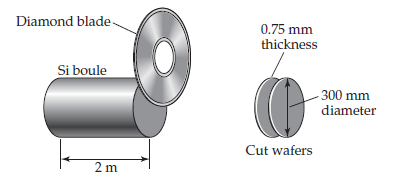# Problem: Silicon for computer chips is grown in large cylinders called “boules” that are 300 mm in diameter and 2 m in length, as shown. The density of silicon is 2.33 g/cm3. Silicon wafers for making integrated circuits are sliced from a 2.0 m boule and are typically 0.75 mm thick and 300 mm in diameter. (a) How many wafers can be cut from a single boule? (b) What is the mass of a silicon wafer? (The volume of a cylinder is given by πr2h, where r is the radius and h is its height.)

🤓 Based on our data, we think this question is relevant for Professor Stoltzfus' class at OSU.

###### Problem Details

Silicon for computer chips is grown in large cylinders called “boules” that are 300 mm in diameter and 2 m in length, as shown. The density of silicon is 2.33 g/cm3. Silicon wafers for making integrated circuits are sliced from a 2.0 m boule and are typically 0.75 mm thick and 300 mm in diameter. (a) How many wafers can be cut from a single boule? (b) What is the mass of a silicon wafer? (The volume of a cylinder is given by πr2h, where r is the radius and h is its height.)